### Highlights#### Visualizing PML

ICERM - September 2016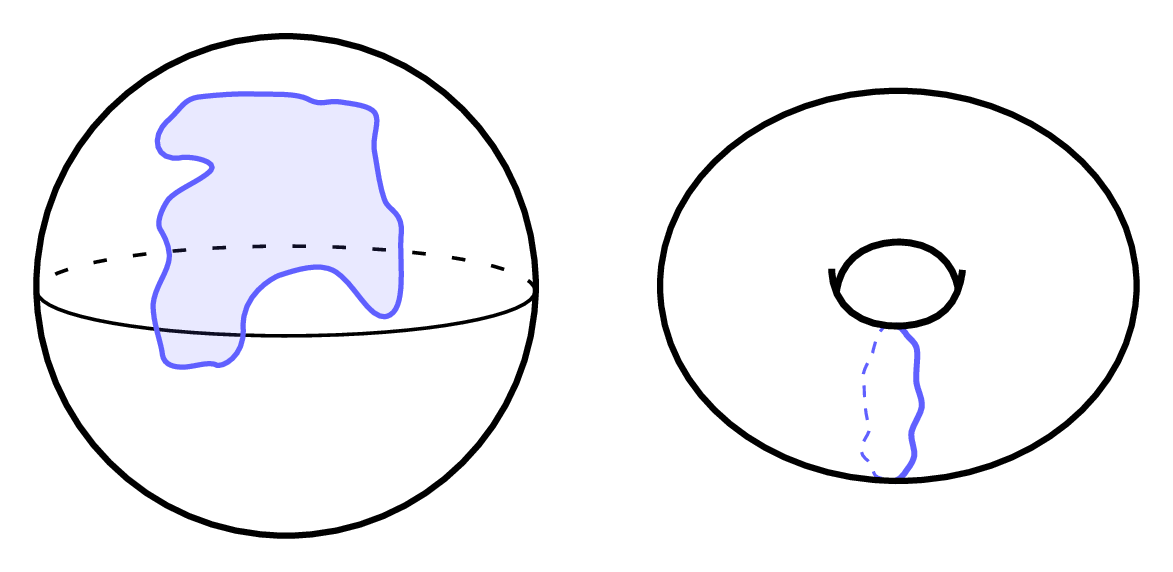Visualizing PML

David Dumas and François Guéritaud

On the surface of a sphere, every simple closed curve (that is, a curve that starts and ends at the same point and which does not cross itself) forms the boundary of a disk , i.e. a contiguous region without holes.  In this sense, the sphere has only one “type” of simple closed curve.  In contrast, on a surface with a more complicated topology—that is, a surface of positive genus —there are infinitely many possible types of simple closed curves that do not bound disks and which cannot be deformed into one another.  The different types are distinguished according to the way a curve winds around and through the handles of the surface.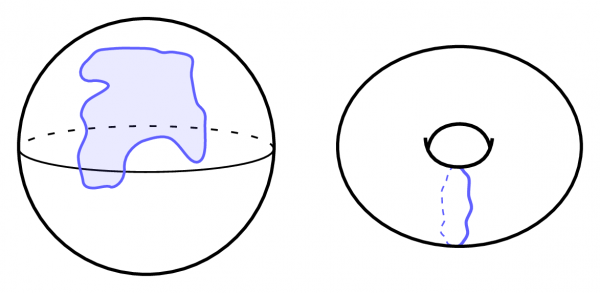Figure 1: Each simple curve on the sphere bounds a region (left); some curves on the torus do not (right).

An important insight of William Thurston in the 1980s was that the set of types of simple closed curves on a given surface can be understood as a geometric object in its own right .  Roughly speaking, to construct this object one represents each type of curve on a surface S of genus g by an abstract point and arranges these so that points are “close” if the corresponding curves spend most of their length winding around the same regions of the surface S in a nearly-parallel manner.  Thurston shows that the resulting collection of points sits densely in a space familiar to mathematicians, namely, the sphere of dimension 6 g -7. This is similar to the way rational numbers (such as 1/2, 2/7, 355/113…) sit densely in the line of real numbers.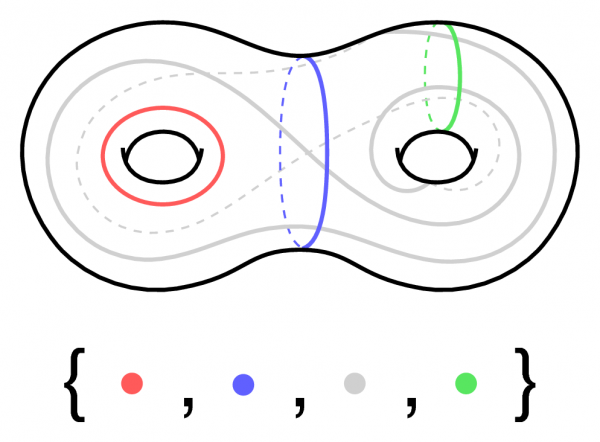Figure 2: Four non-trivial simple curves on a genus two surface S correspond to four points in PML(S).

Thurston’s original constructions of this abstract, high-dimensional sphere (called PML(S), abbreviating “Projective Measured Laminations”), all involved some arbitrary choices: essentially, one adds to the surface some diagram or scaffolding to help organize and catalog the types of simple closed curves.  In a 1986 paper , however, Thurston also described an alternative construction of PML(S) using hyperbolic geometry .  Specifically, he showed that a set of coordinates arising very naturally from the perturbational study of lengths of simple closed curves in a hyperbolic surface S will realize PML(S) as the boundary of a convex body in (6 g -6)-dimensional Euclidean space.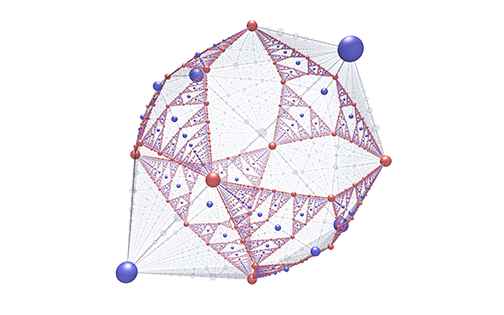Figure 3: Thurston’s convex model of PML for the Möbius strip with two holes.

As Thurston shows in , the shape of this realization of PML(S) is intimately connected to the geometry of Teichmüller space (the 6 g -6-dimensional space of possible hyperbolic models for S) and specifically to the question of understanding the amount of “stretch” that is necessary to move between different hyperbolic models.

The PML Visualization Project  seeks to elucidate the structure of PML(S) and the geometry of Teichmüller space—both of which are topics of ongoing mathematical research—using computer visualizations of Thurston’s convex model for PML(S).  We focus on cases where the dimension is small enough to allow meaningful pictures.  By considering non-orientable surfaces and surfaces with holes, we find a handful of cases in which the dimension of PML(S) is one, two, or three.  We create pictures and animations of these spaces in which the points corresponding to a finite (but very large) collection of simple closed curves are indicated with colored dots.  We use bigger dots to represent shorter curves, so that the picture becomes a simultaneous reflection of the structure of PML(S) and of the surface S itself.  In case PML(S) has dimension three, a further technique (stereographic projection) is used to “open” the 3-dimensional sphere and flatten it into the more familiar 3-dimensional Euclidean space.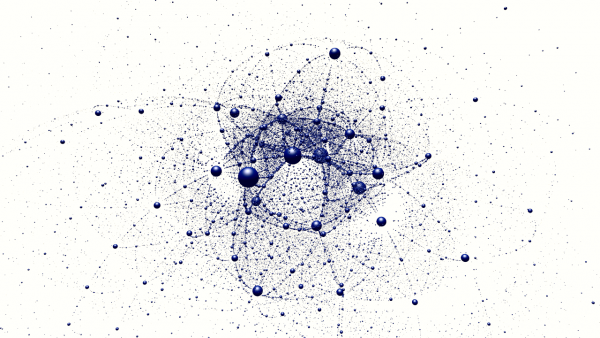Figure 4: Thurston’s convex model of PML for the five-holed sphere, after stereographic projection.

While many properties of the space PML(S), its subset of simple closed curves, and Thurston’s embedding have been established in the last thirty years, it is only in the last few years that these embeddings have been “seen” through computer visualization.  The resulting pictures and animations provide striking illustrations of the intricate self-similar structure of these lamination spaces, and implicitly, of finer structure inherent in the topology of surfaces.

The methods and results of the PML Visualization Project were presented in lectures at the Summer 2016 topical workshop “ Illustrating Mathematics ” at ICERM .

References

 Thurston, William (1980), The geometry and topology of three-manifolds, Princeton lecture notes. http://library.msri.org/books/gt3m/

 Thurston, William (1986).  “Minimal stretch maps between hyperbolic surfaces”. Preprint. http://arxiv.org/abs/math/9801039

 Dumas, David and Guéritaud, François, “The PML Visualization Project”. http://dumas.io/PML

 “Illustrating Mathematics”. Topical workshop at ICERM, June 27-July 1, 2016. http://icerm.brown.edu/topical_workshops/tw16-1-im/